Open in App
Not now

# Label-based indexing to the Pandas DataFrame

• Difficulty Level : Easy
• Last Updated : 25 Oct, 2020

Indexing plays an important role in data frames. Sometimes we need to give a label-based “fancy indexing” to the Pandas Data frame. For this, we have a function in pandas known as pandas.DataFrame.lookup(). The concept of Fancy Indexing is simple which means, we have to pass an array of indices to access multiple array elements at once.

pandas.DataFrame.lookup() function takes equal-length arrays of row and column labels as its attributes and returns an array of the values corresponding to each (row, col) pair.

Syntax: DataFrame.lookup(row_labels, col_labels)

Parameters:
row_labels – The row labels to use for lookup.
col_labels – The column labels to use for lookup.

Returns:
numpy.ndarray

Example 1:

## Python3

 `# importing pandas library``import` `pandas as pd`` ` `# Creating a Data frame``df ``=` `pd.DataFrame([[``'1993'``, ``'x'``, ``5``, ``4``, ``7``, ``2``], ``                   ``[``'1994'``, ``'v'``, ``10``, ``1``, ``2``, ``0``],``                   ``[``'1995'``, ``'z'``, ``2``, ``1``, ``4``, ``12``],``                   ``[``'1996'``, ``'y'``, ``2``, ``1``, ``10``, ``1``], ``                   ``[``'1998'``, ``'x'``, ``2``, ``10``, ``40``, ``12``],``                   ``[``'1999'``, ``'x'``, ``5``, ``8``, ``11``, ``6``]], ``                  ``columns``=``(``'Year'``, ``'Alpha'``, ``'x'``, ``'y'``, ``'z'``, ``'v'``))`` ` `# Display Data frame``df`

Output: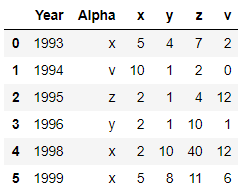## Python3

 `# Use concept of fancy indexing to make new ``# column 'Value' in data frame ``# with help of dataframe.lookup() function``df[``'Value'``] ``=` `df.lookup(df.index, df[``'Alpha'``])`` ` `# Modified Data frame``df`

Output: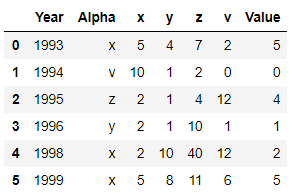In the above example, we use the concept of label based Fancy Indexing to access multiple elements of data frame at once and hence create a new column ‘Value‘ using function dataframe.lookup()

Example 2:

## Python3

 `# importing pandas library``import` `pandas as pd`` ` `# Creating a Data frame``df ``=` `pd.DataFrame([[``'1993'``, ``'Avi'``, ``5``, ``41``, ``70``, ``'Bob'``], ``                   ``[``'1994'``, ``'Cathy'``, ``10``, ``1``, ``22``, ``'Cathy'``], ``                   ``[``'1995'``, ``'Cathy'``, ``24``, ``11``, ``44``, ``'Bob'``], ``                   ``[``'1996'``, ``'Bob'``, ``2``, ``11``, ``10``, ``'Avi'``], ``                   ``[``'1998'``, ``'Avi'``, ``20``, ``10``, ``40``, ``'Avi'``],``                   ``[``'1999'``, ``'Avi'``, ``50``, ``8``, ``11``, ``'Cathy'``]],``                  ``columns``=``(``'Patients'``, ``'Name'``, ``'Avi'``, ``'Bob'``, ``'Cathy'``, ``'Aname'``))`` ` `# Display Data frame``df`

Output: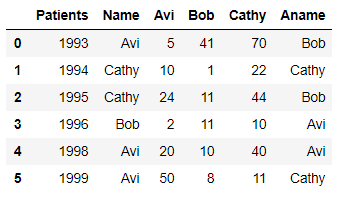## Python3

 `# Use concept of fancy indexing to make two``# new columns in data frame with help of``# dataframe.lookup() function``df[``'Age'``] ``=` `df.lookup(df.index, df[``'Name'``])``df[``'Marks'``] ``=` `df.lookup(df.index, df[``'Aname'``])`` ` `# Modified Data frame``df`

Output: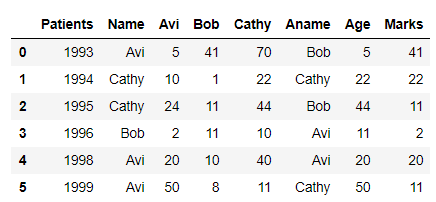In the above example, we use the concept of label based Fancy Indexing to access multiple elements of data frame at once and hence create two new columns ‘Age‘ and ‘Marks‘ using function dataframe.lookup()

Example 3:

## Python3

 `# importing pandas library``import` `pandas as pd`` ` `# Creating a Data frame``df ``=` `pd.DataFrame([[``'Date1'``, ``1850``, ``1992``,``'Avi'``, ``5``, ``41``, ``70``, ``'Avi'``],``                   ``[``'Date2'``, ``1896``, ``1950``, ``'Cathy'``, ``10``, ``1``, ``22``, ``'Avi'``], ``                   ``[``'Date2'``, ``1900``, ``1920``, ``'Cathy'``, ``24``, ``11``, ``44``, ``'Cathy'``], ``                   ``[``'Date1'``, ``1889``, ``1960``, ``'Bob'``, ``2``, ``11``, ``10``, ``'Bob'``], ``                   ``[``'Date2'``, ``1910``, ``1952``, ``'Avi'``, ``20``, ``10``, ``40``, ``'Bob'``],``                   ``[``'Date1'``, ``1999``, ``1929``, ``'Avi'``, ``50``, ``8``, ``11``, ``'Cathy'``]], ``                  ``columns``=``(``'Year'``, ``'Date1'``, ``'Date2'``, ``'Name'``, ``'Avi'``, ``                           ``'Bob'``, ``'Cathy'``, ``'Alpha'``))`` ` `# Display Data frame``df`

Output: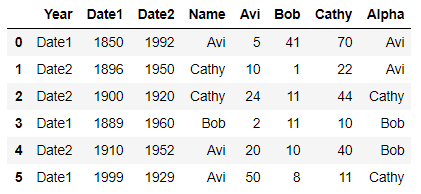## Python3

 `# Use concept of fancy indexing to make two ``# three columns in data frame with help of``# dataframe.lookup() function``df[``'Age'``] ``=` `df.lookup(df.index, df[``'Name'``])``df[``'Height'``] ``=` `df.lookup(df.index, df[``'Alpha'``])``df[``'Date_of_Birth'``] ``=` `df.lookup(df.index, df[``'Year'``])``     ` `# Modified Data frame``df`

Output: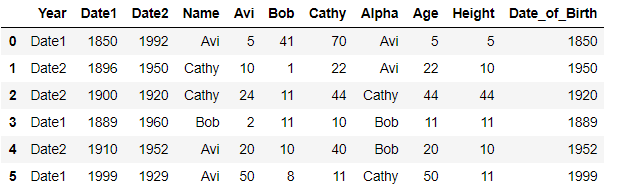In the above example, we use the concept of label based Fancy Indexing to access multiple elements of the data frame at once and hence create two new columns ‘Age‘, ‘Height‘ and ‘Date_of_Birth‘ using function dataframe.lookup()

All three examples show how fancy indexing works and how we can create new columns using fancy indexing along with the dataframe.lookup() function.

My Personal Notes arrow_drop_up